# Multi-digit subtraction worksheets

## Subtraction and regrouping

These subtraction worksheets range from simple subtraction of 2-digit numbers without regrouping to subtraction of large numbers in columns with multiple regroupings. Emphasis is on practicing the regrouping algorithm.

We also have subtraction facts worksheets and thousands of math worksheets by grade level.

## Subtraction without regrouping

2-3 digit minus 1-digit (no regrouping)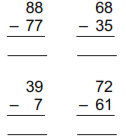2-digit minus 2-digit (no regrouping)
3-digit minus 3-digit (no regrouping)
4-digit minus 4-digit (no regrouping)

## Subtraction with regrouping

2-digit minus 1-digit subtraction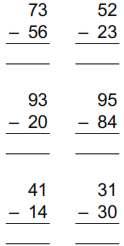2-digit subtraction
3-digit minus 2-digit subtraction
3-digit subtraction
Subtract up to 4-digits
Subtract up to 5-digits
Subtract up to 8-digits

## Horizontal subtraction

Horizontal subtraction (no regrouping)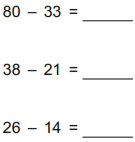Horizontal subtraction
Horizontal subtraction (2 digits)
Horizontal subtraction (3 digits)

## Subtraction tables

Subtraction tables (1-digit subtrahends)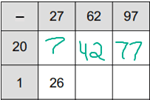Subtraction tables (2-digit subtrahends)

## Missing minuends or subtrahends

Subtraction with missing numbers (no regrouping)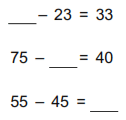Subtraction with missing numbers
Subtraction with missing numbers (2-digits)
Subtraction with missing numbers (3 digits)

## Tens, hundreds and thousands

Subtracting from 10, 100 and 1,000 100 - 61 =
Subtracting from multiples of 10 90 - 61 =
Subtracting from multiples of 100 300 - 187 =
Subtracting from multiples of 1,000 9,000 - 2,832 =
Subtracting across zeros 1,006 - 95 =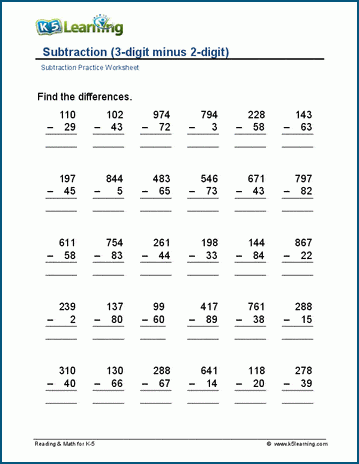Sample subtraction worksheet

What is K5?

K5 Learning offers free worksheets, flashcards and inexpensive workbooks for kids in kindergarten to grade 5. Become a member to access additional content and skip ads.